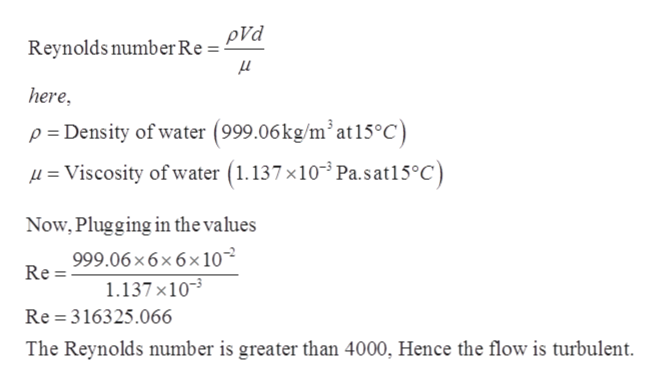# Problem 3: Water at a temperature of 15 °C is flowing steadily in a 6 cm diameter, 25-meter long pipe at an average velocity of 6.0 m/sec. The flow is fully developed. The cross-section is circular. Determine the following for the following wall surface condition i.e. the wall is smooth plastic; a) The Reynolds number. State whether a laminar or turbulent flow is expected.b) The Darcy friction factor, ?, and then find the pressure drop over the length of the pipe.c) Find the corresponding head loss, and calculate the pressure drop over the length of pipe if the velocity is halved, to 3.0 m/sec for the smooth wall case.

Question
14 views

Problem 3: Water at a temperature of 15 °C is flowing steadily in a 6 cm diameter, 25-meter long pipe at an average velocity of 6.0 m/sec. The flow is fully developed. The cross-section is circular. Determine the following for the following wall surface condition i.e. the wall is smooth plastic;
a) The Reynolds number. State whether a laminar or turbulent flow is expected.
b) The Darcy friction factor, ?, and then find the pressure drop over the length of the pipe.
c) Find the corresponding head loss, and calculate the pressure drop over the length of pipe if the velocity is halved, to 3.0 m/sec for the smooth wall case.

check_circle

Step 1

Given:

The temperature of water T = 15oC

The diameter of pipe d = 6 cm = 0.06 m

The length of the pipe L = 25 meter

The wall is smooth plastic. The flow is fully developed. The cross-section of the pipe is circular.

Step 2

The Reynold’s number can be calculated ashelp_outlineImage TranscriptionclosepVd Reynolds number Re = μ here p =Density of water (999.06kg/m2at15°C) Viscosity of water (1.137x10 Pa.sat15°C) Now, Plugging in the values 999.06x6x6x10 Re 1.137 x10 Re 316325.066 The Reynolds number is greater than 4000, Hence the flow is turbulent. fullscreen
Step 3

For the turbulent flow, the Darcy’s friction fact...

### Want to see the full answer?

See Solution

#### Want to see this answer and more?

Solutions are written by subject experts who are available 24/7. Questions are typically answered within 1 hour.*

See Solution
*Response times may vary by subject and question.
Tagged in

### Fluid Mechanics